# VI. Analysis of the 2MASS Second Incremental Release Catalogs

## 3. Color Transformations for the 2MASS Second Incremental Data Release

### a. Summary

Transformation equations are presented to convert colors and magnitudes measured in the AAO, ARNICA, CIT, DENIS, ESO, LCO (Persson standards), MSSSO, SAAO, and UKIRT photometric systems to the photometric system inherent to the 2MASS Second Incremental Data Release (IDR2). The transformations have been derived by comparing 2MASS photometry with published magnitudes and colors for stars observed in these systems. Transformation equations have also been derived indirectly for the Bessell & Brett and Koornneef homogenized photometric systems.

A postscript copy of the paper with complete details on these results is Carpenter (2001, AJ, 121, 2851).

### b. Introduction

One difficulty in directly comparing 2MASS photometry with other near-infrared observations is that these comparison data will often be obtained with a set of filters that have different transmissions profiles and effective wavelengths than the filters adopted for the 2MASS survey. Variations in the filter transmission characteristics can lead to systematic differences in the observed stellar colors, especially for objects with extremely red spectral energy distributions or unusual spectral line features. Any detailed comparisons between 2MASS data and observations conducted at other telescopes then requires that both sets of photometry be placed on a common photometric system. Since 2MASS will provide photometry for sources over the entire sky, it is natural to adopt the 2MASS photometric system as the reference point for these comparisons.

Even though the IDR2 Catalogs are considered preliminary, as all data will be reprocessed at the completion of the 2MASS observations with a final version of the data reduction pipeline, the data already meets a high degree of global photometric uniformity. It is therefore appropriate to determine the color transformations between the IDR2 and other photometric systems in common use today. These transformation equations were derived using 2MASS observations of the standard stars defining current photometric systems. This exercise necessarily emphasizes recent grids of faint near-infrared standards which have effectively replaced more traditional standards that are usually too bright to be observed with modern instrumentation.

### c. The 2MASS Photometric System

i. Filters

Both the Northern and Southern 2MASS telescopes are outfitted with a similar set of optics, filters, and detectors to observe the J, H, and Ks (``K-short'') bands simultaneously (see Section III.1b). Since the total transmission through the atmosphere and the optical elements essentially define any photometric system, it is instructive to review these characteristics as they pertain to 2MASS and note any substantial differences from other photometric systems. Figure 1 shows the transmission as a function of wavelength through the 2MASS optical path, including the telescope mirror reflectivity, the dewar window, anti-reflection coatings, dichroics, filters, and the NICMOS detector quantum efficiency, but excluding the atmosphere. The dominant source of transmission loss through the optical path is the detector quantum efficiency, which is about 0.6-0.65 in the J, H, and Ks bandpasses. A model atmospheric transmission for the mean conditions at Mt. Hopkins is shown separately in Figure 1 as indicated by the thin solid line. The atmospheric transmission data, kindly provided by Martin Cohen, was computed using the USAF PLEXUS code and binned to a resolution of 0.002 micron for display purposes. The transmission curves for both the optical elements and the atmosphere are tabulated in Section III.1b. The primary distinction between 2MASS and many other photometric systems is that the 2MASS Ks filter transmission was specially designed to cut off at 2.3 micron in order to reduce the noise contribution from the thermal background. In this manner, the noise in the Ks band observations will be less sensitive to variations in the ambient temperature, allowing for a more uniform photometric survey. A similar filter has been adopted by the DENIS survey and also was incorporated into the standard star observations by Persson et al. (1998, AJ, 116, 2475). By comparison, more traditional Johnson K filters have significant transmission out to 2.4 microns. We therefore distinguish the 2MASS Ks filter from the Johnson K filter when presenting the color transformations.

As shown in Figure 1, the short and long wavelength cutoff of the 2MASS J filter extends into the atmospheric water absorption features at 1.1 micron and 1.4 micron. In dry weather, the transmission at these wavelengths can be significant compared to typical conditions, implying that the effective J band wavelength, calibration zero-points, and possibly color transformations will depend on the atmospheric water vapor content. Indeed, the J band calibration zero-points often show smooth variations within a night as large as 0.1 magnitudes, and seasonal variations in the average zero-point as large as 0.2 magnitudes are observed. While the 2MASS survey does not record the atmospheric water vapor content directly, these zero-point variations are presumably due to changes in the amount of water vapor.

The above discussion indicates that various aspects of 2MASS photometry need to be investigated before deriving the color transformations between 2MASS and other photometric systems, namely,

• the temporal stability of the 2MASS photometric system over the 3+ years of survey operations;
• the effects of the atmospheric water vapor content on the color transformations, particular those involving J band;
• any differences in the photometric systems between the Northern and Southern surveys.
Nikolaev et al. (2000, AJ, 120, 3340) have discussed these issues in terms of the global magnitude calibration of the 2MASS survey and found that any temporal changes in the global calibration of the J, H, and Ks magnitudes in the 2MASS survey are less than about 0.01 mag. However, since most of the standard stars analyzed by Nikolaev et al. (2000) span a small range of colors, the stability of the 2MASS photometric system as pertaining to the stellar colors remains to be established. The following subsections present such an analysis, and it is shown that any internal variations in the 2MASS color transformations are less than the photometric uncertainties for any individual star.

ii. Temporal Stability

To examine any possible temporal variations in the observed stellar colors, 2MASS photometry in a 1° × 6 ° region near the Galactic plane was analyzed that has been observed on two occasions with the Mt. Hopkins telescope, once on June 12, 1997 near the start of Northern survey operations, and again on May 24, 2000. Thus comparison of these sets of observations will indicate any change in the photometry over nearly a 3-year time period. Besides the long time baseline, this field was chosen since the J-band calibration zero-point on the two nights is the same to within 0.01 mag, suggesting that the atmospheric conditions were similar for both sets of observations (see above). A similar sized region with large differences in the J-band zero-points is analyzed in the following section.

Sources were selected from both survey nights that satisfied the following criteria: (1) the source is free of any flags from the 2MASS data processing pipeline that indicate the photometry may be contaminated by a nearby star; (2) if the magnitudes were computed using PSF fitting photometry, the reduced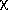2 from the PSF fit is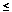15 in each of the J, H, and Ks bands were matched with a source in the second observational set using a 1" search radius. A total of 34,083 sources were matched in this manner. The absolute value of the average photometric offset between the two nights, computed by averaging the difference in photometry for all matched sources, is 0.025, 0.001, and 0.002 mag at J, H, and Ks band respectively. Measured relative to the zero-point calibration uncertainties, the offsets are 0.8, 0.04, and 0.09. Therefore, any difference in the photometric zero-points between the two nights is within the nightly zero-point uncertainties. The zero-point offsets were removed by adding a constant to the first night of observations such that the following analysis compares only the difference the stellar colors. No offsets have been applied to the 2MASS data, however, when comparing the 2MASS photometry with other photometric systems.

Linear fits to the J-K, J-H, and H-K colors between the two sets of observations were performed using the FITEXY program (Press et al. 1992, Numerical Recipes in C) that incorporates uncertainties from both measurements. The reduced2 is about 0.7 for each of the fits, indicating that the colors are well modeled by a linear relation and that the residuals are consistent with random noise. Based on the fit, the difference in the observed colors between observations taken about 3 years apart is less than 0.01 mag for J-H < 1.3 and J-Ks < 1.8. The results for the H-Ks fit are not meaningful since the observed dispersion in the colors (0.089 mag) is only slightly larger than the expected dispersion (0.066 mag) due to photometric noise, such that there is little intrinsic variations in the H-Ks colors for this field. Nevertheless, the strong similarity between the J-H and J-Ks colors suggest that any intrinsic variations in the H-Ks colors must also be small. Thus any temporal changes in the 2MASS color system as judged from the J-H and J-Ks results are comparable to the minimum photometric uncertainties (about 0.02 mag) for any individual star.

iii. Atmospheric Conditions

As discussed above, the effective transmission through the 2MASS J band filter depends on the amount of atmospheric water vapor. Variations in the water vapor content may lead to changes in the J band zero-point calibration and possible introduce additional color terms for the survey data. To quantify this effect, we compared 2MASS photometry for another 1x6 sq. deg. region that was observed on 2 occasions by the Northern survey telescope in which the J band zero-point between the 2 nights differed by 0.16 mag, presumably due to differences in atmospheric water vapor content. A total of 49,389 stars were identified between the two observations using the criteria described above. The average photometric offsets between the two observations measured relative to the zero-point calibration uncertainties are 0.5, 1.1, and 1.6for J, H, and Ks, respectively. Thus the photometric offsets were consistent within the calibration uncertainties, and the zero-points differences were removed before comparing the near-infrared colors (see above). The reduced2 is about 0.9 for each of the linear fits to the near-infrared colors, again indicating that the correlation between the two sets of observations is well represented by a linear relation. Based on the linear fit, the difference in the observed colors between the two observations is less than 0.014 mag for J-H < 3.2 mag, less than 0.021 for J-Ks < 4.7, and less than 0.01 mag for H-Ks < 1.5. Thus no significant change relative to the photometric uncertainties for an individual star was found in the 2MASS photometric system in varying atmospheric conditions.

iv. Comparison between the 2MASS South and North Surveys

While much effort has been placed on making the Northern and Southern operations as identical as possible, small differences in the color systems between the two surveys may still potentially exist. Any differences in the observed stellar colors between the northern and southern hemisphere surveys have been evaluated using 5 fields with declinations between -9 deg and +3 deg that were observed by both telescopes between November 1998 and July 2000. A total of 155,011 point sources were matched between the northern and southern survey data using the criteria described above. The average photometric offset, where the offset is in the sense of the magnitude observed in the north minus the magnitude observed from the south, ranges from -0.011 to 0.039 mag among the three bands. Measured relative to the nightly 1-zero-point calibration uncertainties, the offsets range from -0.45to 1.9. These photometric zero-point offsets for the 5 fields are within the uncertainties of the nightly calibration, and the offsets have been applied to the northern data on a night-by-night basis. Figure 2 compares the observed J-Ka, J-H, and H-Ks colors from the northern and southern survey telescopes. In each figure, the contours represent the density of points in the particular diagram, and the dashed line indicates the expected relation if the near-infrared colors are equal. The derived linear relation between the two observations are

 (J-Ks)North = (1.000 ± 0.001) (J-Ks)South + (-0.001 ± 0.001) (J-H)North = (1.002 ± 0.001) (J-H)South + (-0.003 ± 0.001) (H-Ks)North = (1.009 ± 0.001) (H-Ks)South + (-0.001 ± 0.001)
where the reduced2 from each of the fits is about 0.9. These equations indicate that the J-Ks and J-H colors from the Northern and Southern surveys are statistically indistinguishable. The slope in the H-Ks transformation, if significant, implies that the maximum H-Ks color difference between the northern and southern survey data over the range of colors shown in Figure 2 is 0.012 mag, which is within the nightly calibration uncertainties for any individual star. Therefore, it is assumed that the 2MASS North and South colors systems are identical in the following analysis.

### d. Color Transformations

Table 1 below summarizes the photometric systems that have been analyzed. Included in the table are the references to the photometry that defines these systems, the number of stars with available 2MASS photometry, and links to the tabular data used to derive the transformations. The standard stars that define the Koornneef (1983) and Bessell & Brett (1988) homogenized photometric systems are saturated in the 2MASS images, and the transformation equations have been derived indirectly. The Bessell & Brett color transformations were derived using the 2MASS-CIT and the Bessell & Brett-CIT transformation equations, and for Koornneef, the 2MASS-SAAO was combined with the Koornneef-SAAO transformation equations derived in Carpenter (2001).

 Photometric System Reference Nstars Tables Notes AAO Allen & Cragg (1983) 4 Table 2 a Elias et al. (1983) 10 Table 3 a ARNICA Hunt et al. (1988) 65 Table 4 Bessell & Brett Bessell & Brett (1988) N/A N/A CIT Elias et al. (1982) 33 Table 5 b Elias et al. (1983) 8 Table 6 a DENIS Epchtein et al. (1999) 190 Table 7 ESO van der Bliek et al. (1996) 56 Table 8 Koornneef Koornneef (1983) N/A Table 9 a LCO Persson et al. (1998) 82 Table 10 Table 11 MSSSO McGregor (1994) 20 Table 12 SAAO Carter (1990) 29 Table 13 a Carter & Meadows (1995) 65 Table 14 c UKIRT Hawarden et al. (2000) 72 Table 15
Notes to Table 1:
a. Assumed photometric uncertainty of 0.02 mag
b. Photometric quality flags
a+ :< 0.005
b  :< 0.007
c  :< 0.010
d  :< 0.015
c. Assumed photometric uncertainty of 0.025 mag
References cited in Table 1:
1. Allen, D. A. & Cragg, T. A. 1983, MNRAS, 203, 777
2. Bessell, M. S., & Brett, J. M. 1988, PASP, 100, 1134
3. Carter, B. S. 1990, MNRAS, 242, 1
4. Carter, B. S. & Meadows, V. S. 1995, MNRAS, 276, 734
5. Elias, J. H., Frogel, J. A., Hyland, A. R., & Jones, T. J. 1983, AJ, 88, 1027
6. Elias, J. H., Frogel, J. A., Matthews, K., & Neugebauer, G. 1982, AJ, 87, 1029
7. Epchtein, N. et al. 1999, A&AS, 349, 236
8. Hawarden, T. et al. 2000, in preparation
9. Hunt, L. K., Mannucci, F., Testi, L., Migliorini, S., Stanga, R. M., Baffa, C., Lisi, F., & Vanzi, L. AJ, 87, 1029
10. Koornneef, J. 1983, A&AS, 51, 489
11. McGregor, P. J. 1994, PASP, 106, 508
12. Persson, S. E., Murphy, D. C., Krzeminski, W., Roth, M., & Rieke, M. J. 1998, AJ, 116, 2475
13. van der Bliek, N. S., Manfroid, J., & Bouchet, P. 1996, A&AS, 119, 547

The color transformations between 2MASS and the photometric systems summarized in Table 1 were derived by making a linear fit between the published standard star photometry (or in the case of DENIS, publicly available catalog data) and the 2MASS observations of these stars. The specific variables included in the linear fit are (Ks)2MASS - Kstd vs. (J-K)std, (J-Ks)2MASS vs. (J-K)std, (J-H)2MASS vs. (J-H)std, and (H-Ks)2MASS vs. (H-K)std, where std, treated as the X-variable, represents the standard star photometry for the appropriate photometric system. The transformation equations were derived using the routine FITEXY (Press et al. 1992) that minimizes the2 between the observations and a straight-line model. The uncertainties in both the 2MASS and published photometry are used to evaluate the2 merit function. After examining the residuals from the fit, sources with large discrepancies between the 2MASS and published photometry were removed and the fit was re-derived. Any sources removed from the analysis are noted in Carpenter (2001). Table 16 summarizes the goodness-of-fit parameters from the linear fit, including the reduced2 of the residuals and the probability (q, 0q1) that the reduced2 can be exceeded by chance for gaussian distributed noise. The larger the value of q, the more likely the residuals are consistent with random noise. Table 16 indicates that with the exception of the DENIS-2MASS fit (see discussion below), the 2MASS and published photometry are reasonably described by a linear relationship and the residuals can be explained by photometric noise. The large reduced2 values indicate that the uncertainties in the fitted parameters for the 2MASS-DENIS transformations are underestimated, perhaps by as much as about sqrt(2). The residuals for the K-band transformations tend to have larger reduced2 values than that for the color transformations, especially for photometric systems that incorporate very red infrared standards. As discussed by Elias et al. (1983; see also Persson et al. 1998) these red standards tend to be near star forming regions and have a greater probability of being variable stars. If any of the red standards do have low amplitude variability, the magnitude transformations will be most affected since the J-, H-, and K-band magnitudes will vary simultaneously and produce smaller color changes.

To emphasize that the derived color transformations are valid only for the colors spanned by the published photometry, Figure 3 shows the range of J-K colors contained in the data analyzed here for each photometric system. The appropriate ranges for the J-H and H-K colors can be obtained from inspection of Figures 4-14. In addition, these transformations equations may not apply for objects that exhibit complex spectral energy distributions (e.g., T dwarfs). For these objects, the transformation equations will be sensitive to the exact spectral features that are within the filter transmission curve.

The results from the linear fits are summarized graphically in Figures 4-14 below. In displaying the results, the data are shown as the difference between the 2MASS and standard star photometry as a function of the standard star photometry in order to emphasize subtle, systematic photometric differences. This implies that the X and Y axes are correlated in the plots, which can create artificial trends with slope of -1.0 if the noise in the data exceeds the dynamic range in colors. This effect was quite apparent in the DENIS-2MASS comparisons since the DENIS data have lower signal to noise typically than the 2MASS photometry. For the DENIS results only, the 2MASS photometry is plotted along the X-axis.

The vertical and horizontal bars in Figures 4-14 indicate the 1-photometric uncertainties, although the uncertainties along the X-axis are often smaller then the symbol size given the dynamic range in the plots. The upper left panel in each figures plots the difference in the K-band magnitudes as a function of the J-K color, and the remaining panels directly compare the J-H, J-K, and H-K colors. The dotted line in the upper portion of each panel shows the derived transformation between the appropriate photometric system and 2MASS. The bottom portion of each panel shows the residuals from the fit, where the horizontal dotted line at zero is drawn for reference.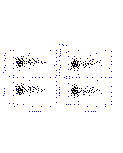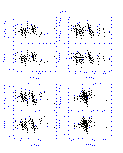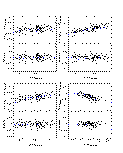Figure 7 Figure 8 Figure 9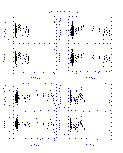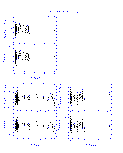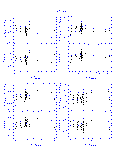Figure 10 Figure 11 Figure 12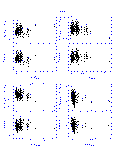Figure 13 Figure 14

### e. Transformation Equations

2MASS - AAO
(Ks)2MASS = KAAO + (-0.032 ± 0.012)+ (-0.021 ± 0.008)(J-K)AAO
(J-H)2MASS = (0.924 ± 0.015)(J-H)AAO+ (0.000 ± 0.016)
(J-Ks)2MASS = (0.943 ± 0.009)(J-K)AAO + (0.024 ± 0.014)
(H-Ks)2MASS = (0.974 ± 0.033)(H-K)AAO + (0.032 ± 0.016)

2MASS - ARNICA
(Ks)2MASS = KARNICA + (0.012 ± 0.006)+ (-0.024 ± 0.011)(J-K)ARNICA
(J-H)2MASS = (1.054 ± 0.020)(J-H)ARNICA + (-0.025 ± 0.008)
(J-Ks)2MASS = (1.056 ± 0.016)(J-K)ARNICA + (-0.018 ± 0.008)
(H-Ks)2MASS = (1.059 ± 0.070)(H-K)ARNICA + (0.007 ± 0.008)

2MASS - Bessell & Brett
(Ks)2MASS = KBB+ (-0.044 ± 0.003)+ (0.000 ± 0.005)(J-K)BB
(J-H)2MASS = (0.980 ± 0.009)(J-H)BB + (-0.045 ± 0.006)
(J-Ks)2MASS = (0.972 ± 0.006)(J-K)BB + (-0.011 ± 0.005)
(H-Ks)2MASS = (0.996 ± 0.019)(H-K)BB + (0.028 ± 0.005)

2MASS - CIT
(Ks)2MASS = KCIT+ (-0.024 ± 0.003)+ (0.000 ± 0.005)(J-K)CIT
(J-H)2MASS = (1.076 ± 0.010)(J-H)CIT + (-0.043 ± 0.006)
(J-Ks)2MASS = (1.056 ± 0.006)(J-K)CIT + (-0.013 ± 0.005)
(H-Ks)2MASS = (1.026 ± 0.020)(H-K)CIT+ (0.028 ± 0.005)

2MASS - DENIS
(Ks)2MASS = (Ks)DENIS+ (-0.024 ± 0.006)+ (0.006 ± 0.004)(J-Ks)DENIS
(J-Ks)2MASS = (0.981 ± 0.006)(J-Ks)DENIS + (0.023 ± 0.009)

2MASS - ESO
(Ks)2MASS = KESO+ (-0.045 ± 0.004)+ (0.005 ± 0.011)(J-K)ESO
(J-H)2MASS = (0.983 ± 0.030)(J-H)ESO + (-0.049 ± 0.008)
(J-Ks)2MASS = (0.956 ± 0.017)(J-K)ESO + (-0.008 ± 0.006)
(H-Ks)2MASS = (0.956 ± 0.126)(H-K)ESO + (0.034 ± 0.006)

2MASS - Koornneef
(Ks)2MASS = KKoornneef+ (-0.047 ± 0.006)+ (0.039 ± 0.019)(J-K)Koornneef
(J-H)2MASS = (1.024 ± 0.024)(J-H)Koornneef + (-0.045 ± 0.006)
(J-Ks)2MASS = (0.970 ± 0.015)(J-K)Koornneef + (-0.017 ± 0.005)
(H-Ks)2MASS = (0.792 ± 0.056)(H-K)Koornneef + (0.027 ± 0.005)

2MASS-LCO
(Ks)2MASS = KLCO+ (-0.006 ± 0.004)+ (-0.001 ± 0.002)(J-K)LCO
(J-H)2MASS = (0.995 ± 0.006)(J-H)LCO + (0.002 ± 0.006)
(J-Ks)2MASS = (1.013 ± 0.005)(J-K)LCO + (-0.007 ± 0.006)
(H-Ks)2MASS = (1.008 ± 0.010)(H-K)LCO + (0.002 ± 0.005)

(Ks)2MASS = (Ks)LCO+ (-0.010 ± 0.004)+ (-0.002 ± 0.002)(J-Ks)LCO
(J-Ks)2MASS = (1.007 ± 0.005)(J-Ks)LCO + (0.002 ± 0.006)
(H-Ks)2MASS = (1.019 ± 0.010)(H-Ks)LCO + (0.005 ± 0.005)

2MASS - MSSSO
(Ks)2MASS = KMSSSO+ (-0.023 ± 0.008)+ (-0.021 ± 0.006)(J-K)MSSSO
(J-H)2MASS = (0.991 ± 0.014) (J-H)MSSSO + (-0.010 ± 0.014)
(J-Ks)2MASS = (1.005 ± 0.008)(J-K)MSSSO + (0.011 ± 0.011)
(H-Ks)2MASS = (1.037 ± 0.029)(H-K)MSSSO + (0.019 ± 0.012)

2MASS - SAAO
(Ks)2MASS = KSAAO+ (-0.025 ± 0.004)+ (0.020 ± 0.007)(J-K)SAAO
(J-H)2MASS = (0.949 ± 0.018)(J-H)SAAO + (-0.054 ± 0.006)
(J-Ks)2MASS = (0.940 ± 0.010(J-K)SAAO + (-0.011 ± 0.005)
(H-Ks)2MASS = (0.961 ± 0.036)(H-K)SAAO + (0.040 ± 0.005)

2MASS - UKIRT
(Ks)2MASS = KUKIRT+ (0.002 ± 0.004)+ (0.004 ± 0.006)(J-K)UKIRT
(J-H)2MASS = (1.069 ± 0.015)(J-H)UKIRT + (-0.027 ± 0.007)
(J-Ks)2MASS = (1.069 ± 0.011)(J-K)UKIRT + (-0.012 ± 0.006)
(H-Ks)2MASS = (1.062 ± 0.027)(H-K)UKIRT + (0.017 ± 0.005)

[Last Updated: 2001 Jan 25 by J. Carpenter (jmc@astro.caltech.edu). Modified 2001 Jan 29 by S. Van Dyk.]

Previous page. Next page.